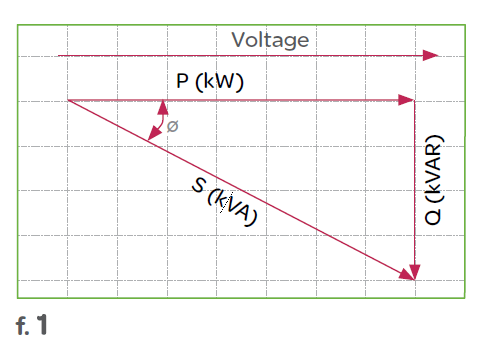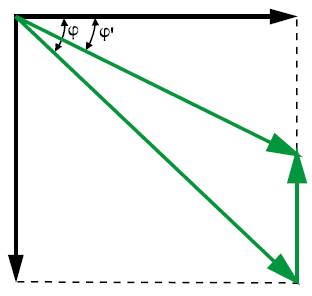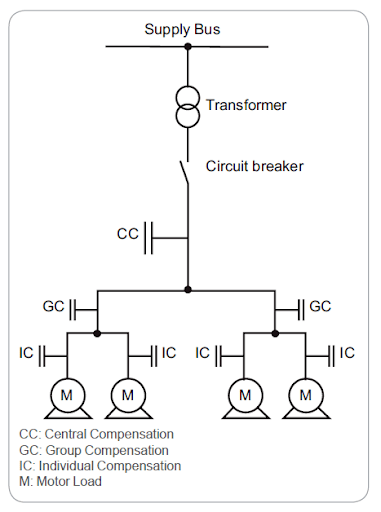# Power factor correction – What is it? Why is it required? How is it achieved?

## Power factor basics:

Power quality is essential for efficient equipment operation, and power factor contributes to this.

Power factor is the measure of how efficiently incoming power is used in an electrical installation. It is the ratio of active to apparent power, when:

• Active Power (P) = the power needed for useful work such as turning a lathe, providing light or pumping water, expressed in Watt or KiloWatt (kW)
• Reactive Power (Q) = a measure of the stored energy reflected to the source which does not do any useful work, expressed in var or Kilovar (kVAR)
• Apparent Power (S) = the vector sum of active and reactive power, expressed in Volt Amperes or in KiloVolt Amperes (kVA)
• The power triangle:Poor power factor (for example, less than 95%) results in more current being required for the same amount of work.

## Power factor correction

Power factor correction (PFC) aims to improve power factor, and therefore power quality. It reduces the load on the electrical distribution system, increases energy efficiency and reduces electricity costs. It also decreases the likelihood of instability and failure of equipment.

Power factor correction is obtained via the connection of capacitors which produce reactive energy in opposition to the energy absorbed by loads such as motors, locally close to the load. This improves the power factor from the point where the reactive power source is connected, preventing the unnecessary circulation of current in the network.## Determining the PFC required

The selection of PFC equipment should be done according to the following four-step process, by persons with the relevant skills:

Step 1: Calculation of the required reactive power

The objective is to determine the required reactive power (Qc (kvar)) to be installed, in order to improve the power factor (cos φ) and reduce the apparent power (S).Qc can be determined from the formula Qc = P (tan φ – tan φ‘), which is deduced from the diagram.

• Qc = power of the capacitor bank in kVAr
• P = active power of the load in kW
• tan φ = tangent of phase shift angle before compensation
• tan φ’ = tangent of phase shift angle after compensation
• The parameters φ and tan φ can be obtained from billing data, or from direct measurement in the installation.

Step 2: Selection of the compensation mode

The location of low-voltage capacitors in an installation can either be central (one location for the entire installation), by sector (section-by-section), at load level, or a combination of the latter two.In principle, the ideal compensation is applied at a point of consumption and at the level required at any moment in time. In practice, technical and economic factors govern the choice.

The location is determined by:

• the overall objective (avoiding penalties on reactive energy, relieving transformers or cables, avoiding voltage drops and sags)
• the operating mode (stable or fluctuating loads)
• the foreseeable influence of capacitors on the network characteristics
• the installation cost
• Step 3: Selection of the compensation type

Different types of compensation should be adopted depending on the performance requirements and complexity of control:

• Fixed, by connection of a fixed-value capacitor bank
• Automatic, by connection of a different number of steps, allowing adjustment of the reactive energy to the required value
• Dynamic, for compensation of highly fluctuating loads
• Step 4: Allowance for operating conditions and harmonics

Operating conditions have a great impact on the life expectancy of capacitors, so the following parameters should be taken into account:

• Ambient temperature (°C)
• Expected over-current related to voltage disturbances, including maximum sustained overvoltage
• Maximum number of switching operations per year
• Required life expectancy
• Some loads (variable speed motors, static converters, welding machines, arc furnaces, fluorescent lamps, etc.) pollute the electrical network by reinjecting harmonics. It is therefore also necessary to consider the effects of these harmonics on the capacitors.

## The benefits of power factor correction

Savings on the electricity bill

Power factor correction eliminates penalties on reactive energy, decreases demand on kVA, and reduces power losses generated in the transformers and conductors of the installation.

Increased available power

Fitting PFC equipment on the low voltage side increases the power available at the secondary of a MV/LV transformer. A high power factor optimises an electrical installation by allowing better use of the components.

Reduced installation size

Installing PFC equipment allows conductor cross-section to be reduced, as less current is absorbed by the compensated installation for the same active power.

Reduced voltage drops

Installing capacitors allows voltage drops to be reduced upstream of the point where the PFC device is connected, therefore preventing overloading of the network and reducing harmonics.